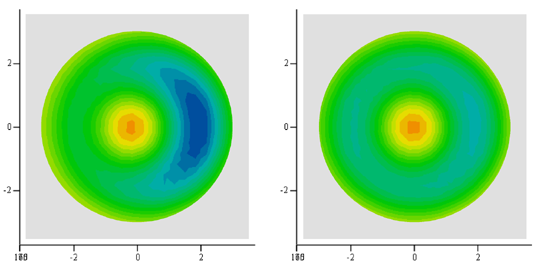Case 6: Aspherical Surface Mapping

This example shows how an aspherical surface, such as that of an aspheric lens, is coated in a planetary-rotation coater. The surface is in the form of "(spherical term) + (r to the 4th power term)". Similar to the study of the spherical surface in Case 5, the surface points in an X-Y grid are imported in the "Surface Coated" panel. Because the lens surface takes a more complex form here, a denser X-Y grid is adopted to include 149 points.

The result shows that the thickness distribution in this case is more complex than the spherical surface. Again, the distribution is asymmetric to the center. By pivoting the surface by -2.0 degrees a more symmetric distribution is obtained. The thickness contour plots before and after pivoting are exhibited below in the left and right column, respectively.

The overall peak-to-valley nonuniformity before and after pivoting is 2.7% and 2.2%, respectively.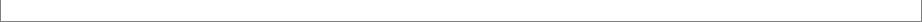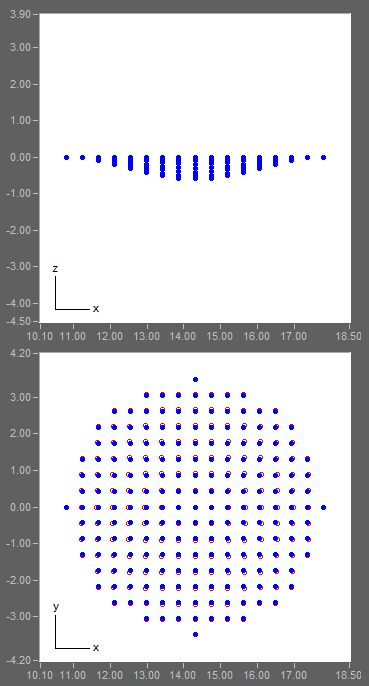Leveled lens
Pivoted lens
maximum=170.42; minimum=163.13
maximum=170.25; minimum=161.26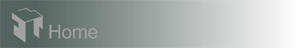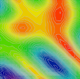Hardware requirements
Request a Free Trial